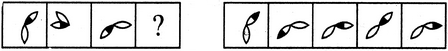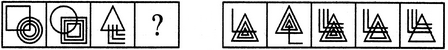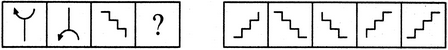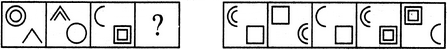# Non Verbal Reasoning - Analogy

## Why Non Verbal Reasoning Analogy?

In this section you can learn and practice Non Verbal Reasoning Questions based on "Analogy" and improve your skills in order to face the interview, competitive examination and various entrance test (CAT, GATE, GRE, MAT, Bank Exam, Railway Exam etc.) with full confidence.

## Where can I get Non Verbal Reasoning Analogy questions and answers with explanation?

IndiaBIX provides you lots of fully solved Non Verbal Reasoning (Analogy) questions and answers with Explanation. Solved examples with detailed answer description, explanation are given and it would be easy to understand. All students, freshers can download Non Verbal Reasoning Analogy quiz questions with answers as PDF files and eBooks.

## Where can I get Non Verbal Reasoning Analogy Interview Questions and Answers (objective type, multiple choice)?

Here you can find objective type Non Verbal Reasoning Analogy questions and answers for interview and entrance examination. Multiple choice and true or false type questions are also provided.

## How to solve Non Verbal Reasoning Analogy problems?

You can easily solve all kind of Non Verbal Reasoning questions based on Analogy by practicing the objective type exercises given below, also get shortcut methods to solve Non Verbal Reasoning Analogy problems.

### Exercise :: Analogy - Section 1

Each of the following questions consists of two sets of figures. Figures A, B, C and D constitute the Problem Set while figures 1, 2, 3, 4 and 5 constitute the Answer Set. There is a definite relationship between figures A and B. Establish a similar relationship between figures C and D by selecting a suitable figure from the Answer Set that would replace the question mark (?) in fig. (D).

1.

Select a suitable figure from the Answer Figures that would replace the question mark (?).(A)     (B)      (C)     (D)                  (1)      (2)      (3)      (4)      (5)

 A. 1 B. 2 C. 3 D. 4 E. 5

Explanation:

2.

Select a suitable figure from the Answer Figures that would replace the question mark (?).(A)     (B)      (C)     (D)                  (1)      (2)      (3)      (4)      (5)

 A. 1 B. 2 C. 3 D. 4 E. 5

Explanation:

The upper element is converted to an element similar to the lower elements and each one of the lower elements is converted to an element similar to the upper element.

3.

Select a suitable figure from the Answer Figures that would replace the question mark (?).(A)     (B)      (C)     (D)                  (1)      (2)      (3)      (4)      (5)

 A. 1 B. 2 C. 3 D. 4 E. 5

Explanation:

The figure gets vertically inverted.

4.

Select a suitable figure from the Answer Figures that would replace the question mark (?).(A)     (B)      (C)     (D)                  (1)      (2)      (3)      (4)      (5)

 A. 1 B. 2 C. 3 D. 4 E. 5

Explanation:

Each one of the upper elements is replaced by an element similar to the lower element(s) and each one of the lower elements is replaced by an element similar to the upper element(s).

5.

Select a suitable figure from the Answer Figures that would replace the question mark (?).(A)     (B)      (C)     (D)                  (1)      (2)      (3)      (4)      (5)

 A. 1 B. 2 C. 3 D. 4 E. 5